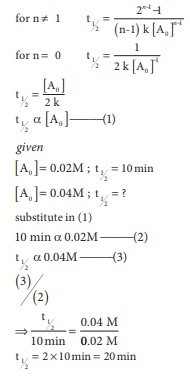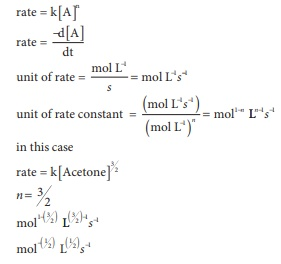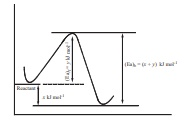# Unit VII: Chemical Kinetics - Online Test

Q1. For a first order reaction A → B the rate constant is x min1 . If the initial concentration of A is 0.01M , the concentration of A after one hour is given by the expression.
Explaination / Solution:In this case

k = x min−1 and [A0] = 0.01M = 1 × 10−2M

t = 1 hour = 60 min

[A]= 1 × 10−2 ( e−60x)

Q2. A zero order reaction X →Product , with an initial concentration 0.02M has a half life of 10 min. if one starts with concentration 0.04M, then the half life is
Explaination / Solution:Q3. Among the following graphs showing variation of rate constant with temperature (T) for a reaction, the one that exhibits Arrhenius behavior over the entire temperature range is
Explaination / Solution:

k = A e –(Ea/RT)

ln k = ln A – (Ea/R)  (1/T)

this equation is in the form of a straight line equatoion

y = c + m x

a plot of lnk vs 1/T is a straight line with negative slope

Q4. For a first order reaction A → product with initial concentration x mol L1 , has a half life period of 2.5 hours . For the same reaction with initial concentration (x/2) mol L1 the half life is
Explaination / Solution:

For a first order reaction

t1/2 = 0.693/k

t1/2 does not depend on the initial concentration and it remains constant (whatever may be the initial concentration)

t1/2 = 2.5 hrs

Q5.

For the reaction, 2NH3 → N2 + 3H2then the relation between k1, k2 and k3 is
Explaination / Solution:(3/2)k1 =  3k2 = k3

1.5 k1 = 3k2 = k3

Q6. The decomposition of phosphine (PH3) on tungsten at low pressure is a first order reaction. It is because the
Explaination / Solution:

At low pressure the reaction follows first order, therefore

Rate α [reactant]1

Rate α ( surface area )

At high pressure due to the complete coverage of surface area, the reaction follows zero order.

Rate α[reactant]0

Therefore the rate is independent of surface area.

Q7. For a reaction Rate = k[acetone]3/2 acetone then unit of rate constant and rate of reaction respectively is
Explaination / Solution:Q8. The addition of a catalyst during a chemical reaction alters which of the following quantities?
Explaination / Solution:

A catalyst provides a new path to the reaction with low activation energy. i.e., it lowers the activation energy.

Q9.

Consider the following statements :

(i) increase in concentration of the reactant increases the rate of a zero order reaction.

(ii) rate constant k is equal to collision frequency A if Ea = 0

(iii) rate constant k is equal to collision frequency A if Ea = °

(iv) a plot of ln(k) vs T is a straight line

(v) a plot of ln (k) vs 1/T  is a straight line with a positive slope.

Correct statements are
Explaination / Solution:

In zero order reactions, increase in the concentration of reactant does not alter the rate. So statement (i) is wrong.

k = A e – (Ea/RT)

if Ea = 0  so, statement (ii) is correct, and statement (iii) is wrong

k = A e0

k = A

ln k = ln A – (Ea /R) (1/ T)

this equation is in the form of a straight line equatoion

y = c + m x

a plot of lnk vs 1/T is a straight line with negative slope

so statements (iv) and (v) are wrong.

Q10. In a reversible reaction, the enthalpy change and the activation energy in the forward direction are respectivelyx kJ mol1 and y kJ mol1 . Therefore , the energy of activation in the backward direction is
Explaination / Solution:(x+y) kJmol-1

(x+y) 103 Jmol-1